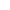搜索
‹‹ 正在移动的网站 ››

▤ 2020-01-21 00:19:20“ 利用线上SCC服务器，在每次执行前上传极小的通信代码，由SCC服务器编译运算再回传，替代了游戏中电脑势力的运算。全过程在一秒内完成，往返数据流量不超过1KB，可以获得20%～60%的执行速度提升。 ”
201.ZU（iOS.223）版本的执行速度优化

 游戏版本 看战斗执行 看海执行 关闭执行动画 变动原因 / 备注 201.ZC 12 + 68 + 20 =100 10 + 32 + 16 = 58 10 + 28 + 16 = 54 号称“最滑版本” 201.ZP 14 + 72 + 25 = 111 14 + 50 + 16 = 80 14 + 36 + 16 = 66 新增了AI外交计谋，导致运算加重 201.ZU 5 + 42 + 4 = 51 4 + 35 + 4 = 43 2 + 15 + 3 = 20 优化了运算算法和重新编译代码

①

②

③

④

 游戏版本 看战斗执行 看海执行 关闭执行动画 变动原因 / 备注 201.ZU【正常】 0 + 5 + 42 + 4 = 51 0 + 4 + 35 + 4 = 43 0 + 2 + 15 + 3 = 20 没有采用线上运算 201.ZU【加速】 1 + 5 + 22 + 4 = 32 1 + 4 + 15 + 4 = 24 1 + 2 + 10 + 3 = 16 采用线上运算

① 阿里云【SCC】服务器价值不菲，很基础的配置都需要月租6500元以上。

②

① 墙裂建议购买前试用，不一定每个设备都有很高的加速效果，领取链接 >>【领取加速试用

② 玩家优惠价格，请玩家登陆后购买，价格在1～10元每月 >> 【购买云加速★★★★★ 4.3